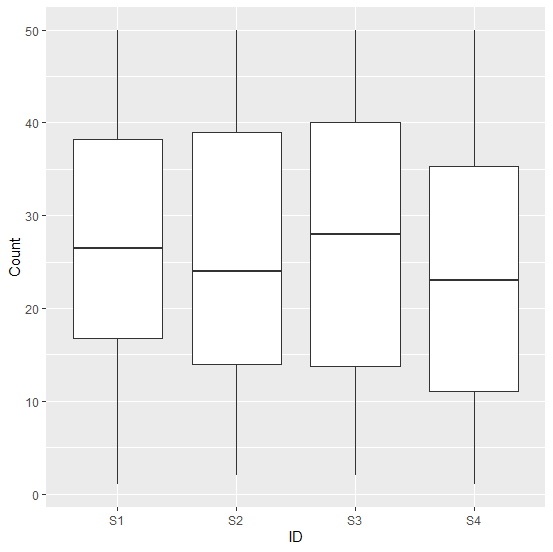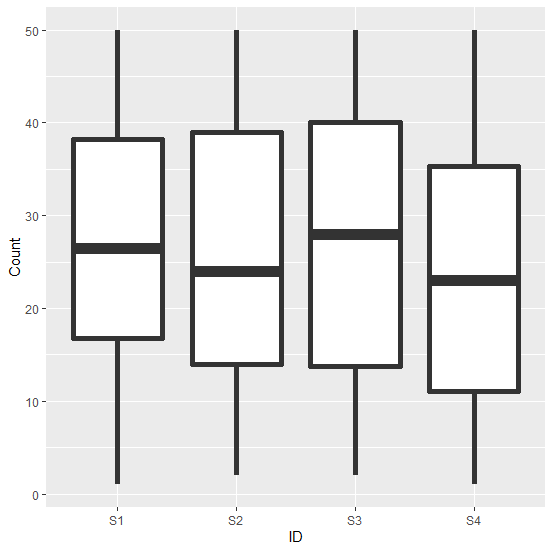# How to increase the width of the lines in the boxplot created by using ggplot2 in R?

When we create a boxplot using ggplot2, the default width of the lines in the boxplot is very thin and we might want to increase that width to make the visibility of the edges of the boxplot clearer. This will help viewers to understand the edges of the boxplot in just a single shot. We can do this by using lwd argument of geom_boxplot function of ggplto2 package.

## Example

Consider the below data frame −

Live Demo

> ID<-rep(c("S1","S2","S3","S4"),times=100)
> Count<-sample(1:50,400,replace=TRUE)
> df<-data.frame(ID,Count)
> head(df,20)

## Output

 ID Count
1 S1 20
2 S2 14
3 S3 17
4 S4 30
5 S1 17
6 S2 23
7 S3 36
8 S4 10
9 S1 14
10 S2 28
11 S3 42
12 S4 10
13 S1 25
14 S2 12
15 S3 4
16 S4 38
17 S1 3
18 S2 45
19 S3 33
20 S4 43

> library(ggplot2)
> ggplot(df,aes(ID,Count))+geom_boxplot()

## OutputCreating the boxplot with different width of the lines −

> ggplot(df,aes(ID,Count))+geom_boxplot(lwd=2)

## Output